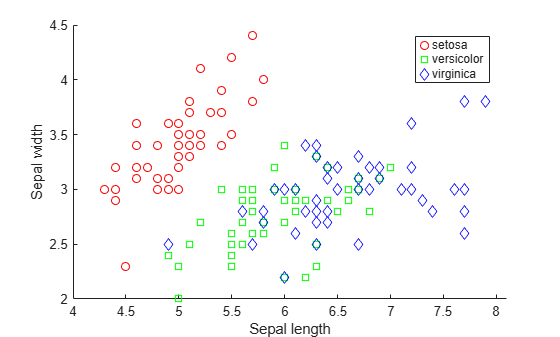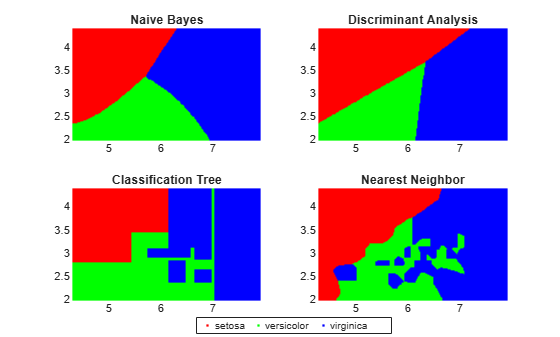Documentation

### This is machine translation

Mouseover text to see original. Click the button below to return to the English version of the page.

## Visualize Decision Surfaces of Different Classifiers

This example shows how to plot the decision surface of different classification algorithms.

```load fisheriris X = meas(:,1:2); y = categorical(species); labels = categories(y);```

`X` is a numeric matrix that contains two petal measurements for 150 irises. `Y` is a cell array of character vectors that contains the corresponding iris species.

Visualize the data using a scatter plot. Group the variables by iris species.

```gscatter(X(:,1),X(:,2),species,'rgb','osd'); xlabel('Sepal length'); ylabel('Sepal width');```Train four different classifiers and store the models in a cell array.

`classifier_name = {'Naive Bayes','Discriminant Analysis','Classification Tree','Nearest Neighbor'};`

Train a naive Bayes model.

`classifier{1} = fitcnb(X,y);`

Train a discriminant analysis classifier.

`classifier{2} = fitcdiscr(X,y);`

Train a classification decision tree.

`classifier{3} = fitctree(X,y);`

Train a k-nearest neighbor classifier.

`classifier{4} = fitcknn(X,y);`

Create a grid of points spanning the entire space within some bounds of the actual data values.

```x1range = min(X(:,1)):.01:max(X(:,1)); x2range = min(X(:,2)):.01:max(X(:,2)); [xx1, xx2] = meshgrid(x1range,x2range); XGrid = [xx1(:) xx2(:)];```

Predict the iris species of each observation in `XGrid` using all classifiers. Plot the a scatter plot of the results.

```for i = 1:numel(classifier) predictedspecies = predict(classifier{i},XGrid); subplot(2,2,i); gscatter(xx1(:), xx2(:), predictedspecies,'rgb'); title(classifier_name{i}) legend off, axis tight end legend(labels,'Location',[0.35,0.01,0.35,0.05],'Orientation','Horizontal')```Each classification algorithm generates different decision making rules. A decision surface can help you visualize these rules.